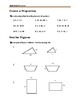# Review of Proportion, Ratio, Percent, Exponents and RadicalsSubject
Resource Type
File Type

PDF

(525 KB|10 pages)
Product Rating
4.0
(1 Rating)
Standards
• Product Description
• StandardsNEW

· Content 100% aligned with the 2019-2020 Standard test

· Provided and tested by Math test experts

· Dynamic design and easy-to-follow activities

· A fun, interactive and concrete learning process

· Targeted, skill-building practices

Understand that rational expressions form a system analogous to the rational numbers, closed under addition, subtraction, multiplication, and division by a nonzero rational expression; add, subtract, multiply, and divide rational expressions.
Rewrite simple rational expressions in different forms; write 𝘢(𝘹)/𝘣(𝘹) in the form 𝘲(𝘹) + 𝘳(𝘹)/𝘣(𝘹), where 𝘢(𝘹), 𝘣(𝘹), 𝘲(𝘹), and 𝘳(𝘹) are polynomials with the degree of 𝘳(𝘹) less than the degree of 𝘣(𝘹), using inspection, long division, or, for the more complicated examples, a computer algebra system.
Know and apply the Binomial Theorem for the expansion of (𝘹 + 𝘺)ⁿ in powers of 𝘹 and y for a positive integer 𝘯, where 𝘹 and 𝘺 are any numbers, with coefficients determined for example by Pascal’s Triangle.
Prove polynomial identities and use them to describe numerical relationships. For example, the polynomial identity (𝘹² + 𝘺²)² = (𝘹² – 𝘺²)² + (2𝘹𝘺)² can be used to generate Pythagorean triples.
Identify zeros of polynomials when suitable factorizations are available, and use the zeros to construct a rough graph of the function defined by the polynomial.
Total Pages
10 pages
Included
Teaching Duration
30 minutes
Report this Resource to TpT
Reported resources will be reviewed by our team. Report this resource to let us know if this resource violates TpT’s content guidelines.